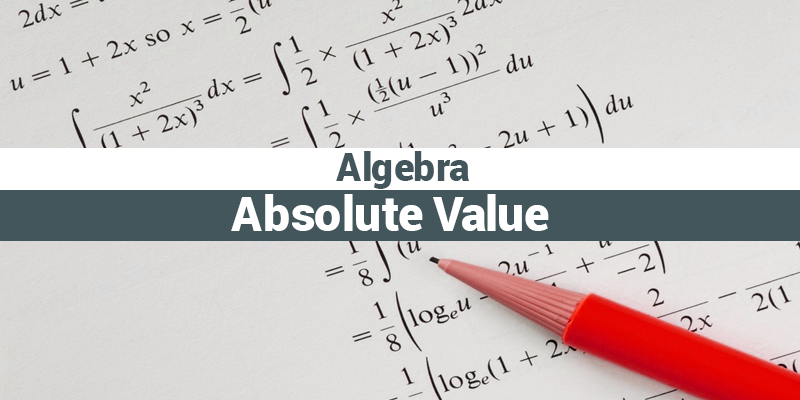# GMAT Quant: Algebra – Absolute ValueWe all have studied absolute value in our schools but with time it became a bit complex. Down the line what we basically learnt in absolute value is:

|positive| = positive

As well as,

|negative| = positive

But, GMAT is never going to ask you such an easy question.

In GMAT Exam, you can expect the following types of questions:

•  |3x + 8| = 42

So, 3x + 8 = 42 or, 3x + 8 = -42

3x = 34        or, 3x = -50

x = 11.333    or,  x = -16.666

Let’s solve some more complex questions and see what GMAT actually tests.

1. Find the value of x in:

|x + 2| + |x – 3| = |2x + 3|

1. -5
2. -2
3. 1
4. 4
5. 7

Solution:

If we suddenly look at this question, we won’t have any idea of solving it.

So, let’s solve it step-by-step.

• There is a variable in this question.
• When a variable is here, we can simply plug-in any number at its place.
• Value of x is one of the given options. So, let’s substitute these options and check which one fulfills the constraints.

|-5 + 2| + |-5 – 3| = |2 (-5) + 3|

Not equal.

|-2 – 2| + |-2 + 3| = |2 (-2) + 3|

Not equal

|1 + 2| + |1 – 3| = |21+3|

Equal

Tip: Solving a problem in traditional way can eat-up a lot of your time. So, try different approaches.

1. If m + |m| = 0, then m is,
2. positive
3. 0
4. Negative

Let’s check each possibility,

Case1. m is positive.

|m| = m

So, m + |m| = 0

m + m = 0

So this case is not possible.

Case2. m is 0

So, |m| = 0

0 + 0 = 0

So this is a possible case

Case3. m is negative.

|m| = -m

|m| + m = 0

-m + m = 0

So, m is either 0 or negative.

We’ll be glad to help you in your GMAT preparation journey. You can ask for any assistance related to GMAT and MBA from us by calling us at +918884544444. You can write to us at gmat@byjus.com.